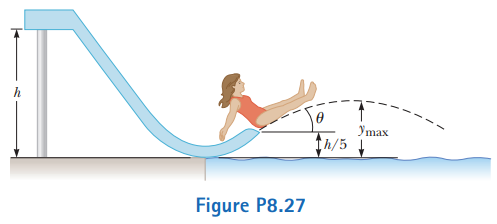# Problem: A child of mass m starts from rest and slides without friction from a height h along a slide next to a pool (Fig.  P8.27). She is launched from a height h/5 into the air over the pool. We wish to find the maximum height she reaches above the water in her projectile motion.(a) Is the child–Earth system isolated or nonisolated? Why?(b)  Is there a nonconservative force acting within the system?(c) Define the configuration of the system when the child is at the water level as having zero gravitational potential energy. Express the total energy of the system when the child is at the top of the waterslide.(d) Express the total energy of the system when the child is at the launching point.(e)  Express the total energy of the system when the child is at the highest point in her projectile motion.(f) From parts (c) and (d), determine her initial speed vi at the launch point in terms of g and h.(g) From parts (d), (e), and(f), determine her maximum airborne height ymax in terms of h and the launch angle θ.(h) Would your answers be the same if the waterslide were not frictionless? Explain.

###### FREE Expert Solution

(a)

A system is isolated when it has a collection of matter which does not interact with the rest of the universe at all.

85% (316 ratings)###### Problem Details

A child of mass m starts from rest and slides without friction from a height h along a slide next to a pool (Fig.  P8.27). She is launched from a height h/5 into the air over the pool. We wish to find the maximum height she reaches above the water in her projectile motion.

(a) Is the child–Earth system isolated or nonisolated? Why?
(b)  Is there a nonconservative force acting within the system?
(c) Define the configuration of the system when the child is at the water level as having zero gravitational potential energy. Express the total energy of the system when the child is at the top of the waterslide.
(d) Express the total energy of the system when the child is at the launching point.
(e)  Express the total energy of the system when the child is at the highest point in her projectile motion.
(f) From parts (c) and (d), determine her initial speed vi at the launch point in terms of g and h.
(g) From parts (d), (e), and
(f), determine her maximum airborne height ymax in terms of h and the launch angle θ.
(h) Would your answers be the same if the waterslide were not frictionless? Explain.Frequently Asked Questions

What scientific concept do you need to know in order to solve this problem?

Our tutors have indicated that to solve this problem you will need to apply the Solving Projectile Motion Using Energy concept. You can view video lessons to learn Solving Projectile Motion Using Energy. Or if you need more Solving Projectile Motion Using Energy practice, you can also practice Solving Projectile Motion Using Energy practice problems.

What professor is this problem relevant for?

Based on our data, we think this problem is relevant for Professor Chow's class at UCF.

What textbook is this problem found in?

Our data indicates that this problem or a close variation was asked in Physics for Scientists and Engineers - Serway Calc 9th Edition. You can also practice Physics for Scientists and Engineers - Serway Calc 9th Edition practice problems.